## JavaScript Loops

When repeating the same task, it becomes cumbersome and hard to maintain.

Let's add 5 items to a box.

``````let i = 1 // initialization
if (i < 5) console.log(`Item \${i}`); i++; // Item 1 (step 1)
if (i < 5) console.log(`Item \${i}`); i++; // Item 2 (step 2)
if (i < 5) console.log(`Item \${i}`); i++; // Item 3 (step 3)
if (i < 5) console.log(`Item \${i}`); i++; // Item 4 (step 4)
if (i <= 5) console.log(`Item \${i}`); // Item 5 (step 5)
``````

The example above is what programmers call WET (We Enjoy Typing or Write Everything Twice) code.

DRY (Don't Repeat Yourself) codes are best practices.

The code snippet above can be rewritten as shown below:

``````for (let i = 1; i <= 5; i++) console.log(`Item \${i}`);
``````

The iterator variable `i` is a conventional name in computer programming but can be named anything like `iter` ... `counter` etc.

An execution of the loop body `{...}` is called an iteration. The loop in the example above makes five (5) iterations.

A loop body is whatever in the curly braces:

``````{ console.log(`Item \${i} }
``````

A single-line body does not require curly braces.

### Increment

An increment is an increase in a number by one.

See the example below (post-increment):

``````let step = 0;
step++; // 0
console.log(step); // 1
step++; // 1
console.log(step); // 2
``````

In the example above, `step++` is called a post-increment. A pre-increment looks like `++step`.

See another example below (pre-increment):

``````let step = 0;
++step; // 1
console.log(step); // 1
++step; // 2
console.log(step); // 2
``````

### Decrement

A decrement is a decrease in a number by one.

See the example below (post-decrement):

``````let step = 2;
step--; // 2
console.log(step); // 1
step--; // 1
console.log(step); // 0
``````

In the example above, `step--` is called a post-decrement. A pre-decrement looks like `--step`.

See another example below (pre-decrement):

``````let step = 2;
--step; // 1
console.log(step); // 1
--step; // 0
console.log(step); // 0
``````

`step++` is the same as `step += 1` or `step = step + 1`

There are two common ways of looping in JavaScript:

• `while` loop;
• `do...while` and
• `for` loop

### While loop

While the condition is truthy, the code from the loop body gets executed.

Syntax:

``````// initialization;
while (condition) {
// step and
// statements
}
``````

See the example below:

``````let i = 0;
while (i < 5) {
i++;
console.log(`Item \${i}`);
}
``````

If `i++` gets omitted, the output will always iterate to be `item 1` forever. Always include the step in a loop!

### do...while loop

The `do...while` loop will always execute at least once even though the condition evaluates to false.

Syntax:

``````// initialization;
do {
// step and
// statements
} while (condition);
``````

See the example below:

``````let i = 0;
do {
i++;
console.log(`Item \${i}`); // Item 1
} while (i > 5);
``````

In the example above, `i` or `0` is not `> 5` in the first iteration. It is a false evaluation, but it still evaluates the statement `Item 1`.

Use the `do...while` syntax only when you want to execute at least once regardless of the condition being truthy.

### For Loop

The `for` loop is a common way of looping.

Syntax:

``````for (initialization; condition; step) {
// statements
}
``````

See the example below:

``````for (let i = 1; i <= 5; i++) {
console.log(`Item \${i}`);
}
``````

The code snippet above is the same as the code below:

``````let i = 0;
for ( ; i < 5; ) {
i++;
console.log(`Item \${i}`);
}
``````

It now looks like a `while` loop!

To create an infinite loop, the code is:

``````let i = 0;
for (;;) {
i < 5;
i++;
console.log(`Item \${i}`);
}
``````

Avoid the example above!### Breaking a loop

We can also `break` a loop if a condition gets satisfied.

The syntax commonly used is shown below:

Syntax:

``````for (initialization; condition; step) {
// statements
if (conditionSatisfied) {
break;
}
}
``````

See the example below:

``````for (let i = 1; i <= 5; i++) {
console.log(`Item \${i}`);
if (i === 3) {
break;
}
}

/*
Output:
Item 1
Item 2
Item 3
*/
``````

Forever iterating of a loop can be prevented by a `break` if a condition is satisfied.

``````let i = 0;
for (;;) {
i < 5;
i++;
console.log(`Item \${i}`);
if (i === 3) {
break;
}
}

/*
Output:
Item 1
Item 2
Item 3
*/
``````

### Continue to the next iteration

A particular iteration in a loop can get skipped with the `continue` keyword.

The syntax commonly used is shown below:

Syntax:

``````for (initialization; condition; step) {
if (conditionSatisfied) {
continue;
}
// statements
}
``````

See the example below:

``````for (let i = 1; i <= 5; i++) {
if (i === 3) {
continue;
}
console.log(`Item \${i}`);
}

/*
Output:
Item 1
Item 2
Item 4
Item 5
*/
``````

More than one iterations can be skipped:

``````for (let i = 1; i <= 5; i++) {
if (i % 2 === 0) {
continue;
}
console.log(`Item \${i}`);
}

/*
Item 1
Item 3
Item 5
*/
``````

### Nested loops

Loops can be nested into the parent loop.

See the example below:

``````for (let i = 1; i <= 5; i++) {
console.log('---------');
for (let j = 1; j <= 10; j++)
console.log(`\${i} x \${j} = \${i * j}`);
}
``````

See how the above example look:

``````iteration 1: 1, 2, ..., 10;
iteration 2: 1, 2, ..., 10;
...   ...   ...
iteration 5: 1, 2, ..., 10;
``````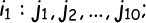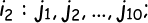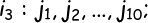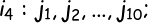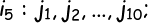### Label

``````outer: for (let i = 1; i <= 5; i++) {
console.log('----------');

for (let j = 1; j <= 10; j++) {
console.log(`\${i} x \${j} = \${i * j}`);
}
if (i === 3)  {
break outer;
}
}
``````

The label `outer` is optional but used to break or continue an iteration.

See another example below:

``````outer: for (let i = 1; i <= 5; i++) {
console.log('----------');

inner: for (let j = 1; j <= 10; j++) {
console.log(`\${i} x \${j} = \${i * j}`);
if (i === 3 && j === 5)  {
break outer;
}
}
}
``````

Happy Coding!!!### TechStack | Bluehost

• Get a website with a free domain name for 1st year and a free SSL certificate.
• 1-click WordPress install and 24/7 support.
• Starting at \$3.95/month.
• 30-Day Money-Back Guarantee.